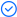WPS Office

Free All-in-One Office Suite with PDF EditorRead, edit, and convert PDFs with the powerful PDF toolkit.Microsoft-like interface, easy to use.

Windows • MacOS • Linux • iOS • Android# How to check if value exists in a range in Excel

August 9, 2022
5.3K Views

While working on excel with lots of data, sometimes you want to check if a certain value exists in a range of data. This might seem a simple task when your range is small and you can check manually that whether the required value exists in range. But when you are required to check a bigger range of data in excel to check if value exists in range WPS (2016/2019/mac/online) then it becomes a very tough task and it might take you ages to perform this task. Luckily there are different shortcuts possible in excel to find if a value exists in range in excel.

This article covers different shortcut methods in excel check if value exists in range WPS (2016/2019/mac/online). Once you go through this article you will be able to easily identify if a value exists in a range.

Three different shortcut ways of checking if a value exists in a range in excel.

1.1 Using COUNTIF function to check if a value exists in range in excel

Among different ways to check if a value exists in a range the first methods is using COUNTIF function. You can use COUNTIF formula to see if a value exists in a range by following simple below mentioned steps and picture illustrations.

Steps:

1.Open WPS Excel /Spreadsheet file where you want to check if a value exists in range in excel.

2.Click on the cell where you want your output to reflect whether a value exists in range.

3.Type “=COUNTIF” and press Tab.COUNTIF Function will be initiated.

4.You need to enter two parameters in this function I.e. Range (Range in which which you want to check if a value exists in it)Criteria (Here you enter the value inside inverted commas I.e. “xyz”)

5.Then you press enter.This function will return the exact number of times the required value exists in the selected range.

1.2 Using COUNTIF embedded in IF function to check if a value exists in range in excel

Another way of checking if value exists in range is by using a COUNTIF function embedded in IF function. You can use this method to see if a value exists in a range by following simple below mentioned steps and picture illustrations.

Steps:

1.Open WPS Excel /Spreadsheet file where you want to check if a value exists in range in excel.2.Click on the cell where you want your output to reflect whether a value exists in range.

3.Type “=IF(COUNTIF” and press Tab.IF Function with embedded COUNTIF Function will be initiated.

4.You need to enter four parameters in this function I.e. Range (Range in which which you want to check if a value exists in it)Criteria (Here you enter the value inside inverted commas I.e. “xyz”)Value IF True (You can use “Yes” here)Value IF False (You can use “No” here)

5.Then you press enter.This function will return whether a value exists in a range in simple yes or no terms instead of exact number of time as shown section 1.1.

1.3 Using MATCH Function embedded in ISNUMBER function to check if a value exists in range in excel

Another method of checking if a value exists in a range is to use MATCH Function Embedded in ISNUMBER function.

Steps:

1.Open WPS Excel /Spreadsheet file where you want to check if a value exists in range in excel.2.Click on the cell where you want your output to reflect whether a value exists in range.

3.Type “=ISNUMBER(MATCH” and press Tab.ISNUMBER Function with embedded MATCH Function will be initiated.

4.You need to enter three parameters in this function I.e. Lookup Value  (value that needs to be checked in a range)Look Up Array (range in which the value is to be checked)Match Type (This must be set to 0 to search for an exact match)

5.Then you press enter.This function will return whether a value exists in a range in simple True or False terms instead of exact number of time as shown section 1.1.

This article has covered the three different shortcut ways to check if a value exists in a range in excel.

Hopefully you have learned How to Excel check if value exists in range WPS (2016/2019/mac/online) ?  If you want to know more about Excel features, you can follow WPS Academy to learn.